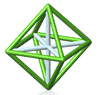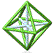Park City Mathematics Institute Investigating Geometry Project Abstract Drafts of Project Files (password required)
Transformation Rumble! (A Board Game)
Robert LaColla*, Sara Rezvi
Students learn best through self-motivating games. In this game, students take turns transforming a polygon of their choosing. However, if the polygon is transformed onto space already occupied by a polygon from someone else or an earlier move, then only the remaining part of the polygon may be graphed. Whoever ends the game with more total area covered by their polygons wins! By interpreting verbal statements into transformation notation, calculating area, and increasing their fluency with transforming polygons in the coordinate plane, the students meet several goals in the CCSS (G-CO5, G-CO4, G-CO2)

Tangramzzzzz
Jen Katz*, Jennifer Outzs, Rebecca Ueno
This project is a one week exploration unit that uses tangram shapes on a coordinate grid to investigate the effects of dilation factors on coordinate points, side lengths, and areas. Our goal was to create an activity meeting the Grade 7 Common Core Standards in the Geometry domain by having students solve problems involving scale drawings of geometric figures, including computing actual lengths and areas from a scale drawing and reproducing a scale drawing at a different scale.

Using Transformations to derive the formula for Axis of Symmetry of Parabolas
Nicole Manning*
In this project students will complete tables of values for families of quadratic equations and graph parabolas. They will look for patterns in the tables of values and relate the table, graph and quadratic equation. They will conjecture and observe how (isolated) changes made to the equation result in transformations on the graph. They will use properties of symmetry and distance (between points on the parabola with equal y values) to plot the axis of symmetry. Then, students will be asked to create, test, and revise a general formula for the axis of symmetry, using their knowledge of the relationship between the quadratic equation and the location of the parabola. Once students find the axis of symmetry using the formula, they will be asked to identify alternate methods of graphing (other than table of values). CCSS content standards F-IF-4, F-IF-7, and F-IF-9 will be addressed as well as Mathematical Practices #1, 3, 5, 6, 7, and 8.

Linear Functions and Geometric Transformations on the Number Plane
Vo Duy Cuong*
By using Dynamic Geometry Software or other tools, students will investigate the transformations which map a free point M(x, y) on the number plane to a point M'(x', y'), where x' = f(x) or/and y' = g(y) with some given linear function f(x) and g(x).

Translations and Shape Properties
Andrea VanDunk*, Sara Rezvi
This project provides a week worth of lesson activities that leads students through the discovery of the properties of rectangles, isosceles triangles, kites, trapezoids, and rhombuses through transformations. Each activity can lead to rich class discussion and include three extension questions designed to deepen, connect, and assess student understanding.

Back to Investigating Geometry IndexPCMI@MathForum Home || IAS/PCMI Home© 2001 - 2018 Park City Mathematics Institute IAS/Park City Mathematics Institute is an outreach program of the Institute for Advanced Study, 1 Einstein Drive, Princeton, NJ 08540 Send questions or comments to: Suzanne Alejandre and Jim King

With program support provided by Math for America

This material is based upon work supported by the National Science Foundation under DMS-0940733 and DMS-1441467. Any opinions, findings, and conclusions or recommendations expressed in this material are those of the author(s) and do not necessarily reflect the views of the National Science Foundation.